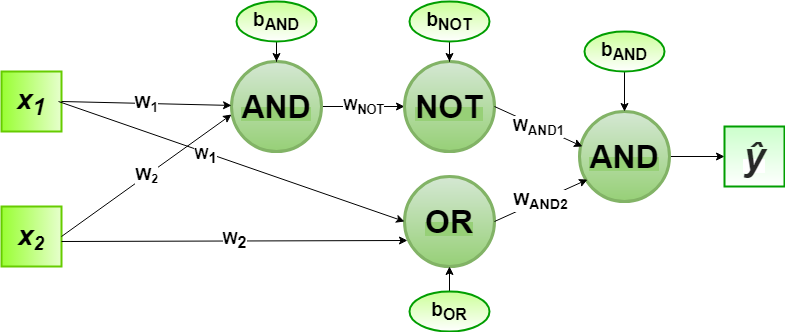Skip to content
Related Articles
Implementation of Perceptron Algorithm for XOR Logic Gate with 2-bit Binary Input
• Last Updated : 13 Jul, 2020

In the field of Machine Learning, the Perceptron is a Supervised Learning Algorithm for binary classifiers. The Perceptron Model implements the following function:For a particular choice of the weight vectorand bias parameter, the model predicts outputfor the corresponding input vector.

XOR logical function truth table for 2-bit binary variables, i.e, the input vectorand the corresponding output000
011
101
110

We can observe that,Designing the Perceptron Network:

1. Step1: Now for the corresponding weight vectorof the input vectorto the AND and OR node, the associated Perceptron Function can be defined as:2. Step2: The outputfrom the AND node will be inputed to the NOT node with weightand the associated Perceptron Function can be defined as:3. Step3: The outputfrom the OR node and the outputfrom NOT node as mentioned in Step2 will be inputed to the AND node with weight. Then the corresponding outputis the final output of the XOR logic function. The associated Perceptron Function can be defined as:For the implementation, the weight parameters are considered to beand the bias parameters are.

Python Implementation:

 # importing Python libraryimport numpy as np  # define Unit Step Functiondef unitStep(v):    if v >= 0:        return 1    else:        return 0  # design Perceptron Modeldef perceptronModel(x, w, b):    v = np.dot(w, x) + b    y = unitStep(v)    return y  # NOT Logic Function# wNOT = -1, bNOT = 0.5def NOT_logicFunction(x):    wNOT = -1    bNOT = 0.5    return perceptronModel(x, wNOT, bNOT)  # AND Logic Function# here w1 = wAND1 = 1, # w2 = wAND2 = 1, bAND = -1.5def AND_logicFunction(x):    w = np.array([1, 1])    bAND = -1.5    return perceptronModel(x, w, bAND)  # OR Logic Function# w1 = 1, w2 = 1, bOR = -0.5def OR_logicFunction(x):    w = np.array([1, 1])    bOR = -0.5    return perceptronModel(x, w, bOR)  # XOR Logic Function# with AND, OR and NOT  # function calls in sequencedef XOR_logicFunction(x):    y1 = AND_logicFunction(x)    y2 = OR_logicFunction(x)    y3 = NOT_logicFunction(y1)    final_x = np.array([y2, y3])    finalOutput = AND_logicFunction(final_x)    return finalOutput  # testing the Perceptron Modeltest1 = np.array([0, 1])test2 = np.array([1, 1])test3 = np.array([0, 0])test4 = np.array([1, 0])  print("XOR({}, {}) = {}".format(0, 1, XOR_logicFunction(test1)))print("XOR({}, {}) = {}".format(1, 1, XOR_logicFunction(test2)))print("XOR({}, {}) = {}".format(0, 0, XOR_logicFunction(test3)))print("XOR({}, {}) = {}".format(1, 0, XOR_logicFunction(test4)))
Output:
XOR(0, 1) = 1
XOR(1, 1) = 0
XOR(0, 0) = 0
XOR(1, 0) = 1


Here, the model predicted output () for each of the test inputs are exactly matched with the XOR logic gate conventional output () according to the truth table.
Hence, it is verified that the perceptron algorithm for XOR logic gate is correctly implemented.My Personal Notes arrow_drop_up